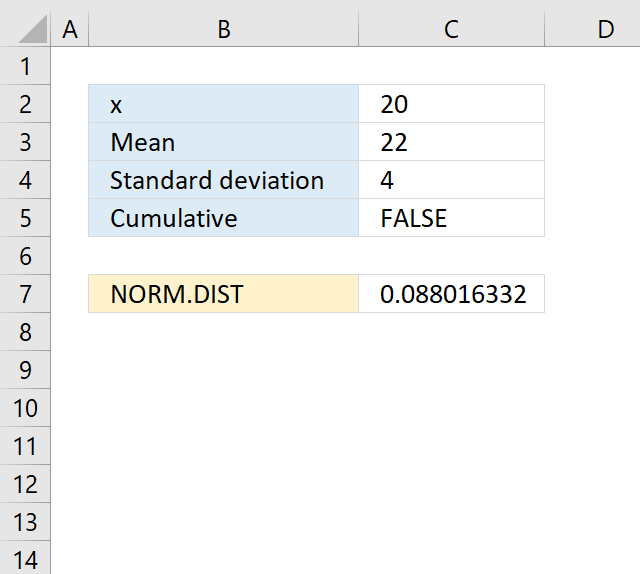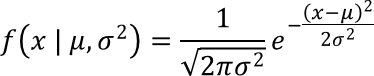Author: Oscar Cronquist Article last updated on April 13, 2018The NORM.DIST function calculates the normal distribution for a given mean and standard deviation.

Formula in cell C7:

=NORM.DIST(C2,C3,C4,C5)

### Excel Function Syntax

NORM.DIST(xmeanstandard_devcumulative)

### Arguments

 x Required. A number to calculate the distribution for. mean Required. The average of the distribution. standard_dev Required. The standard deviation of the distribution. cumulative Required. A boolean value that determines which distribution the NORM.DIST function returns. TRUE - Cumulative distribution FALSE - Probability mass function

The NORM.DIST function is very useful if you are working with statistics. Here is how the function works in detail (cumulative = FALSE).Use the AVERAGE function to calculate the arithmetic mean, used in the second argument in the NORM.DIST function.

How to use the AVERAGE function

Calculates the average of numbers in a cell range. In other words, the sum of a group of numbers and […]

Use the STDEV.P function to calculate the standard deviation used in the third argument.

How to use the STDEV.P function

The STDEV.P function returns standard deviation based on the entire population. The standard deviation is how widely numbers are distributed […]

If mean = 0, standard_dev = 1, and cumulative = TRUE, the standard normal distribution is returned.

If cumulative = TRUE, the formula is calculated the integral from negative infinity to x.